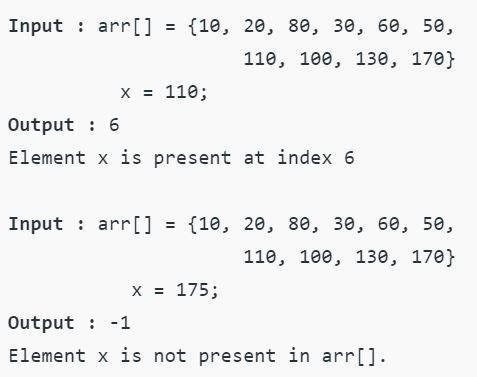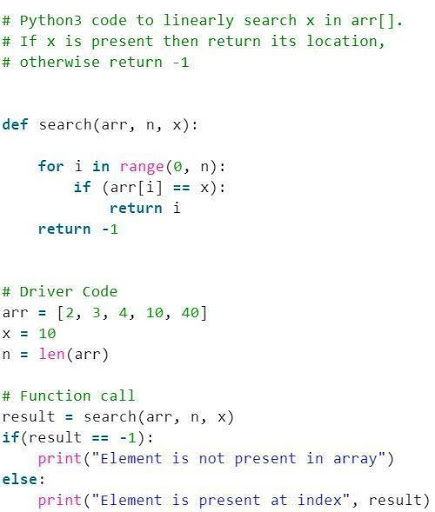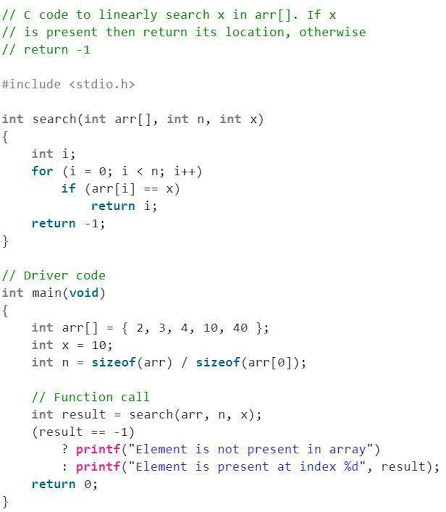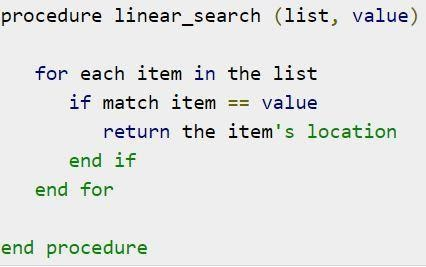# Introduction to Linear Search Algorithm: Introduction & Features[With Examples]

## What is Searching?

Searching is the process of finding a given element in a list of elements. It helps in the searching of a particular record. Therefore, it is a technique of identifying the place of a given item. The success of a searching process depends on whether the item to be searched has been identified or not.

The Data structure allows the searching of data through two methods.

1. Linear search or sequential search
2. Binary search

## Linear Search

Linear search algorithms are a type of algorithm for sequential searching of the data. This algorithm finds a given element with O(n) complexity. It is applied to a collection of items. Each and every item of the data is searched sequentially, and returned if it matches the searched element. If no matches are found, then the search keeps on continuing till the end of the collected data. It is basically a technique of exploring every element while traversing the list. The searching algorithm can be applied to both the sorted and the unsorted data. Practically linear search is rarely used because of the faster searching options provided by other search algorithms like binary search algorithms and hashtables.

### Steps in the linear search algorithm

• Reading of the search element by the user.
• The element to be searched is compressed with the first element of the list.
• If the elements match, then a return is generated.
• If the elements don’t match then the element to be searched is compared to the second element of the list.
• The process is repeated until the element is matched.

### Features of linear search algorithms

1. It is usually applied to a small list of unsorted or unordered data.
2. Time is linearly dependent on the number of elements, therefore having a time complexity if O(n).
3. The implementation is very simple.

### Linear search algorithms

A continuous looping method goes on unless and until the item is found

• algorithm Seqnl_Search(list, item)
• Pre: list != ;
• Post: return the index of the item if found, otherwise: 1
• index <- fi
• while index < list.Cnt and list[index] != item //cnt: counter variable
• index <- index + 1
• end while
• if index < list.Cnt and list[index] = item
• return index
• end if
• return: 1
• end Seqnl_Search

### Example of linear search

Problem: Given an array arr[] of n elements, write a function to search a given element x in arr[].

Figure 1: An example of code showing the implementation of linear search algorithmSource

Linear search algorithms can be used in several programming languages.

1. #### Linear search in Python

Figure 2: An example of code showing a linear search algorithm in Python languageSource

Output: Element is present at index 3

1. #### Linear search in C

Figure 3: An example of code showing a linear search algorithm in C languageSource

Output: Element is present at index 3

1. Linear search in Data structure

Pseudocode for a linear search problem in Data structure is

Figure 4: The pseudocode for linear search algorithmSource

## Binary Search

Binary search is an algorithm to search elements in an array of elements. Compared to the linear search algorithm, the binary search algorithm is applied to a sorted list of data.

Binary search algorithm includes the following steps

• Comparison of the element to be searched with the element from the middle of the list or array.
• If the element matches with the element from the list, it returns the index of the middle element.
• If no match is returned, it is checked whether the element is greater than or lesser than the element in the middle.
• For an element of greater value than the middle element, the search is carried on the right side of the array.
• Similarly, if the element is lesser in value than the middle element, then the search is carried on to the left side of the list.

Therefore, binary search is best applied when the data contains large number rod elements in a sorted manner. This makes it a requisite for the search algorithm that the list/array should be sorted.

### Features of Binary Search

• The binary search algorithm is useful for searching a large number of elements in an array.
• The binary search algorithm has a time complexity of O(logn).
• Implementation of a binary search algorithm is simple.

### Binary Search Algorithm

• Algorithm Binary_Search(list, item)
• Set L to 0 and R to n: 1
• if L > R, then Binary_Search terminates as unsuccessful
• else
• Set m (the position in the mid element) to the floor of (L + R) / 2
• if Am < T, set L to m + 1 and go to step 3
• if Am > T, set R to m: 1 and go to step 3
• Now, Am = T,
• the search is done; return (m)

Example of Binary Search

Figure 5: An example of code showing the implementation of binary search algorithmSource

Output:

Element is present at index 3

## Conclusion

In this article, we have looked into what is a Linear Search Algorithm and also studied in detail how to search for a certain element from a list using the Linear Search Algorithm. Lastly, we also saw how we could practically implement the Linear Search Algorithm using Python 3 as a language and get our desired output.

If you are interested in Data Science, you must check out IIIT-B and upGrad’s Executive PG Program in Data Science which has been carefully crafted for working professionals and offers numerous case studies, hands-on workshops, 1-on-1 mentorship, and much more.

### How is linear search different from binary search?

The following illustrates the major differences between linear search and binary search:
Linear Search -
1. Elements need not be in any specific order for linear search.
2. In Linear search, elements are sequentially accessed.
3. O(n), where n is the number of array elements.
4. Linear Search is preferred when the data set is relatively smaller.
Binary Search -
1. Elements must be sorted for binary search.
2. Elements are randomly accessed in binary search.
3. O(log n), where n is the number of array elements.
4. Binary Search is generally preferable for a larger data set.

### What are the applications of linear searching?

The following are some of the significant applications of linear search:
Linear search is efficient for searching in data sets having a smaller number of elements. If only a single search is needed to be performed in unordered data, then the linear search is preferable over other searching algorithms.
Searching a node in a linked list becomes efficient when a linear search is performed. Furthermore, binary search and linear search have the same time complexities in linked lists. Binary Search can even get complex for performing search operations in linked lists.
If the elements in the data set are modified repeatedly, then in such cases linear search is the preferred choice.

### Give examples where linear search can be seen in real life?

The linear search algorithm is analogous to real-life searching. There are several examples that prove this:
Searching for a book in a pile of 100 books. You will linearly scan the name of each book until you find the right one
Finding your cab in the parking lot. When you book a cab ride, you have the license plate number of the cab. To find your cab, the obvious method would be to match every car’s license plate with your number.
Finding your favourite cookies on the store shelves. From a huge collection of cookies in a store, you will search every row one by one.## 0 replies on “Introduction to Linear Search Algorithm: Introduction & Features[With Examples]”